#### 期刊菜单

Analysis of Power Flow Characteristics of Flexible Floating Raft Isolation System
DOI: 10.12677/IJM.2021.104027, PDF, HTML, XML, 下载: 163  浏览: 220

Abstract: As a common vibration control method for modern ship equipment, the floating raft vibration iso-lation system is an important technology for vibration isolation and noise reduction, and power flow is an effective method to evaluate the vibration isolation effect. Based on the power flow theory, the finite element model of the flexible floating raft vibration isolation system is established. The vibration isolation effect of the floating raft vibration isolation system is studied, and the visualization path of vibration energy transmission of the floating raft vibration isolation system is revealed. The effects of isolator parameters including isolator stiffness, isolator damping and equipment mass on the isolation effect of floating raft vibration isolation system are studied. The results show that the finite element power flow analysis method can provide a basis for the structural design and safe operation of floating raft vibration isolation system.

1. 引言

20世纪90年代初，国内开始研究浮筏隔振系统，提出了很多方法并发表了相关论文。江国和等  以系统固有频率和筏架振动响应为依据，研究隔振器刚度和筏架质量对隔振性能的影响；Sun等  研究了具有弹性基础的浮筏隔振系统半有源、有源隔振问题；张树桢等  采用子结构分析法进行柔性浮筏隔振系统的理论建模和隔振性能研究；李增光  采用阻抗导纳法研究了激励间相位差对输入基座功率流的影响。本文在柔性基础隔振理论研究基础上，建立了柔性浮筏隔振系统模型，利用ABAQUS及其二次开发功能对其进行稳态振动功率流分析，揭示了振动能量在系统中传递的一般特性，并分析了基础刚度、隔振器刚度、机器质量、隔振器安装位置以及隔振器和柔性基础阻尼对隔振效果的影响。

2. 板壳的功率流理论

$\left\{\begin{array}{l}{\sigma }_{x}=\frac{E}{1-{\mu }^{2}}\left(\frac{{\partial }^{2}w}{\partial {x}^{2}}+\mu \frac{{\partial }^{2}w}{\partial {y}^{2}}\right)\\ {\sigma }_{y}=\frac{E}{1-{\mu }^{2}}\left(\frac{{\partial }^{2}w}{\partial {y}^{2}}+\mu \frac{{\partial }^{2}w}{\partial {x}^{2}}\right)\\ {\tau }_{xy}=\frac{E}{1+\mu }\frac{{\partial }^{2}w}{\partial xy}\end{array}$ (1)

v——沿y方向上的变形；

w——沿z方向上的变形；

$\mu$ ——泊松比；

E——抗拉弹性模量。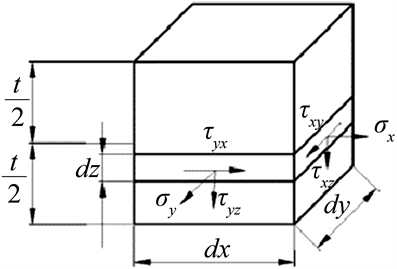Figure 1. Micro-element stress of plate and shell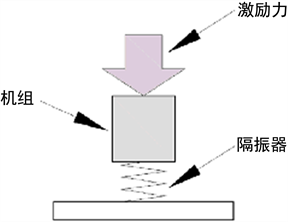(a) 单层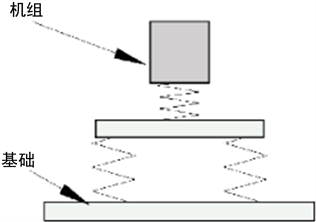(b) 双层

Figure 2. Schematic diagram of vibration isolation system

${N}_{x}={\int }_{-h/2}^{h/2}{\sigma }_{x}\text{d}z=\frac{Eh}{1-{\mu }^{2}}\left(\frac{\partial u}{\partial x}+\mu \frac{\partial v}{\partial y}\right)$ (2)

${N}_{y}={\int }_{-h/2}^{h/2}{\sigma }_{y}\text{d}z=\frac{Eh}{1-{\mu }^{2}}\left(\frac{\partial v}{\partial y}+\mu \frac{\partial u}{\partial x}\right)$ (3)

${N}_{xy}={\int }_{-h/2}^{h/2}{\tau }_{xy}\text{d}z=\frac{Eh}{2\left(1-\mu \right)}\left(\frac{\partial u}{\partial y}+\frac{\partial v}{\partial x}\right)$ (4)

${M}_{x}={\int }_{-h/2}^{h/2}{\sigma }_{x}z\text{d}z=-D\left(\frac{{\partial }^{2}w}{\partial {x}^{2}}+\mu \frac{{\partial }^{2}w}{\partial {y}^{2}}\right)$ (5)

${M}_{y}={\int }_{-h/2}^{h/2}{\sigma }_{y}z\text{d}z=-D\left(\mu \frac{{\partial }^{2}w}{\partial {x}^{2}}+\frac{{\partial }^{2}w}{\partial {y}^{2}}\right)$ (6)

${M}_{xy}={M}_{yx}={\int }_{-h/2}^{h/2}{\tau }_{xy}z\text{d}z=-D\left(1-\mu \right)\frac{{\partial }^{2}w}{\partial x\partial y}$ (7)

${Q}_{x}={\int }_{-h/2}^{h/2}{\tau }_{xz}\text{d}z=-D\left(\frac{{\partial }^{3}w}{\partial {x}^{3}}+\frac{{\partial }^{3}w}{\partial x\partial {y}^{2}}\right)$ (8)

${Q}_{y}={\int }_{-h/2}^{h/2}{\tau }_{yz}\text{d}z=-D\left(\frac{{\partial }^{3}w}{\partial {x}^{2}\partial y}+\frac{{\partial }^{3}w}{\partial {y}^{3}}\right)$ (9)

${p}_{x}=-\frac{\omega }{2}Im\left\{{N}_{x}{u}_{x}^{\ast }+{N}_{xy}{u}_{y}^{\ast }+{Q}_{x}{u}_{z}^{\ast }+{M}_{x}{\theta }_{y}^{\ast }+{M}_{xy}{\theta }_{x}^{\ast }\right\}$ (10)

${p}_{y}=-\frac{\omega }{2}Im\left\{{N}_{xy}{u}_{x}^{\ast }+{N}_{y}{u}_{y}^{\ast }+{Q}_{y}{u}_{z}^{\ast }+{M}_{yx}{\theta }_{y}^{\ast }+{M}_{y}{\theta }_{x}^{\ast }\right\}$ (11)

3. 浮筏隔振系统功率流分析

3.1. 浮筏隔振系统介绍

3.2. 浮筏结构的有限元模型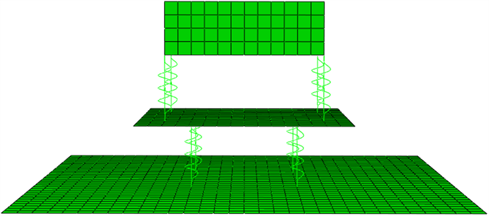Figure 3. Finite element model of floating raft system

3.3. 浮筏结构功率流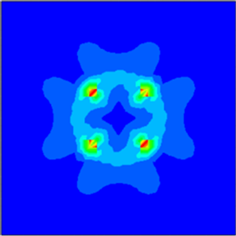Figure 4. Power flow diagram and power flow vector of floor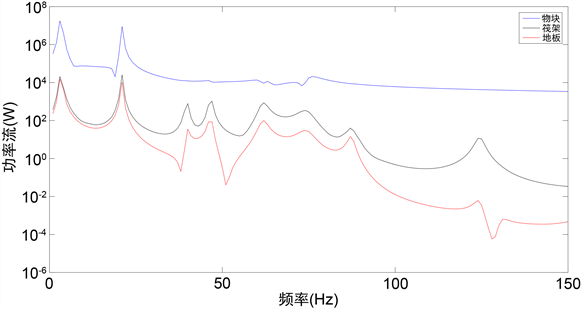Figure 5. The amount of power contained in each component at different frequencies

4. 浮筏隔振系统振动传递特性分析

4.1. 激励力大小对隔振效果的影响Figure 6. The effect of the incentive force on the power flow

4.2. 系统结构参数对隔振效果的影响Figure 7. Influence of the upper stiffness on the power flow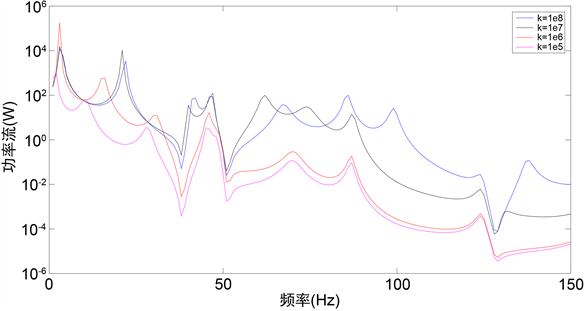Figure 8. Influence of stiffness of lower isolator on power flow of input base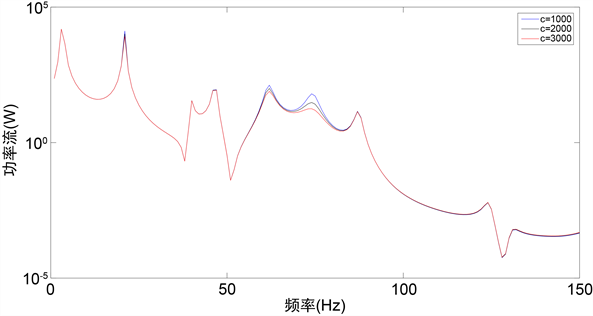Figure 9. Influence of damping coefficient of upper isolator on power flow of input baseFigure 10. Influence of damping coefficient of lower vibration isolator on power flow of input base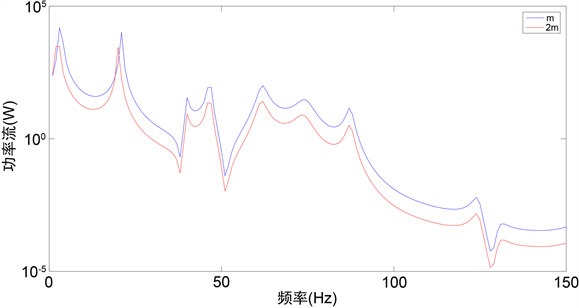Figure 11. Impact of device quality on the power flow of the input base

5. 总结

1) 在浮筏隔振系统中，物块、筏架、地板的总功率依次减小，也就是通过隔振器后能量越来越少。从整体趋势上看频率越大传递到筏架和地板的功率越小，激励力的激振频率越大其隔振效果越好。

2) 在保证隔振系统稳定性以及其他限制条件的前提下，调整隔振器的安装位置、减小隔振器刚度，增加机器的质量可以减小传递到基础的功率流，从而提高浮筏隔振系统的隔振效果。

3) 增大隔振器的阻尼可以减小共振峰处的功率流大小，使机器通过共振峰的振动不至于太过强烈，但是增大阻尼不能提高浮筏隔振系统的隔振效果。

  高立冬, 喻浩, 王暖. 减振降噪技术的应用设计[J]. 中国造船, 2002, 43(2): 44-49.  李新德, 宋孔杰, 孙玉国. 复杂激励下平置板式浮筏功率流传递特性研究[J]. 应用力学学报, 2003, 20(2): 32-37.  许树浩, 桂洪斌. 浮筏系统隔振性能的功率流评价指标[J]. 船舶力学, 2012, 16(5): 567-572.  朱翔, 李天匀, 赵耀, 等. 损伤圆柱壳的振动功率流特性研究[J]. 船舶力学, 2007, 11(2): 284-292.  江国和, 邵文政. 三维弹性浮筏隔振系统参数对隔振性能的影响[J]. 机电信息, 2010(18): 184-185.  Sun, H.L., Zhang, K., Zhang, P.Q., et al. (2010) Application of Dynamic Vibration Absorbers in Floating Raft System. Applied Acoustics, 71, 250-257. https://doi.org/10.1016/j.apacoust.2009.09.005  张树桢, 陈前. 柔性浮筏隔振系统的理论建模与仿真研究[J]. 船舶力学, 2012, 16(10): 1187-1198.  李增光. 浮筏及双层隔振装置隔振性能计算与分析[J]. 噪声与振动控制, 2015, 35(6): 65-68.  Sciully, D. and Inman, D.J. (1998) Isolation Design for a Flexible System. Journal of Sound and Vibration, 216, 251-267. https://doi.org/10.1006/jsvi.1998.1667  Tkins, P.A.A., Wright, J.R. and Worden, K. (2000) An Extension of force Appropriation to the Identification of Non-Linear Multi-Degree Offreedom Systems. Journal of Sound and Vibration, 237, 23-43. https://doi.org/10.1006/jsvi.2000.3033  Xiong, Y.P. and Song, K.J. (1996) Power Flow Analysis for a New Isolation System-Flexible Floating Raft. Chinese Journal of Mechanical Engineering, 9, 260-264.  江国和, 沈荣瀛, 等. 舰船机械设备隔振技术研究进展[J]. 船舶力学, 2006, 10(1): 135-144.